# scipy.spatial.distance.matching¶

scipy.spatial.distance.matching(u, v)

Computes the Matching dissimilarity between two boolean n-vectors u and v, which is defined as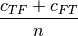where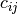is the number of occurrences of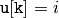and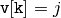for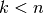.

Parameters : u : ndarray An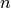-dimensional vector. v : ndarray An-dimensional vector. d : double The Matching dissimilarity between vectors u and v.

#### Previous topic

scipy.spatial.distance.mahalanobis

#### Next topic

scipy.spatial.distance.minkowski